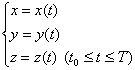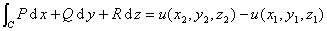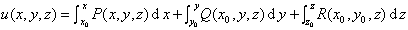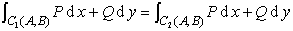[对弧长的曲线积分]  若函数f(x,y,z)在光滑曲线C:的各点上有定义并且连续（图6.6）则[对坐标的曲线积分]  若函数P=P(x,y,z),Q=Q(x,y,z),R=R(x,y,z)在光滑曲线C:[全微分的情形]  若函数P=P(x,y,z),Q=Q(x,y,z),R=R(x,y,z)在区域V中的任一条光滑曲线C上连续，并且在单连通区域V内有连续的一阶偏导数的函数P,Q,R能表成全微分[格林公式]

1°曲线积分与二重积分的关系.C为逐段光滑的简单（无自交点）闭曲线，围成单连通的有界区域S,这围线的方向使区域S保持在左边，若函数P(x,y),Q(x,y)及它们的一阶偏导数在S+C上连续，则有格林公式2° 曲线积分与积分线路的关系.若函数P,Q,在区域S上连续，且